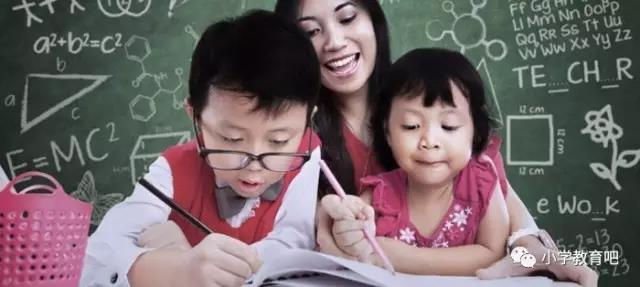# 小学数学+-x÷的秘密你都知道吗？超快口算技巧教给你！

二维码 2391、口诀

2、例题
1376+98=1474 计算方法：1376+100-2
3586+898=4484 计算方法：3586+1000-102
5768+9897=15665 计算方法：5768+10000-103

1、口诀

2、例题
47+74=121 计算方法：（4+7）x 11=121
68+86=154 计算方法：（6+8）x 11=154
58+85=143 计算方法：（5+8）x 11=143

1、口诀

2、例题
365427158
644785963
+742334452
———————
1752547573

1、例题
321-98=223

8135-878=7257

91321-8987= 82334

2、总结

1、例题
74-47=27

83-38=45

92-29=63

2、总结

1、例题
936-639=297

723-327=396

873-378=495

2、总结

1、例题
73-27=46

613-387=226

8112-1888=6224

2、总结

1、口诀

2、例题
67x 63= 4221

7x3=21写在42的后面，即为乘积4221
38x32=1216

8x2=16写在12的后面，即为乘积1216
76x74=5624

6x4=24写在56的后面，即为乘积5624
81 x89=7209

1x9=09写在72的后面，（未满10补零）即为乘积7209

1.口诀

2.例题
76x 36＝2736

6x6= 36写在27的后面，即乘积2736
68x 48＝3264

8x8=64写在32的后面，即为乘积3264
54x54=2916

4x4=16写在29的后面，即为乘积2916
83 x 23=1909

3x3=09（未满10补零）写在19的后面，即为乘积1909

57的平方是5x5+7+7x7=3249
58的平方是5x5+8+8x8=3364........

1、例题
37x66=2442

7x6=42写在24的后面，即乘积2442
46 x77=3542

6x7=42写在35的后面，即乘积3542
44x28=1232

4x8=32写在12的后面，即乘积1232
88888888888
x 37
————————

7x8=56 （尾积）

3288888888856

2、总结

1、例题

2、口诀

1、例题
13x12=156

3x2=6 150+6=156
15x17=255

5x7=35 220+35=255
18 x16=288

8x6=48 240+48=288
19x18=342

9x8=72 270+72=342

2、口诀

1、例题
31x21=651

1x1=1
51 x71=3621

5+7=12（写2进1） 1x1=1
61 x81=4941

6+8=14（写4进1） 1x1=1
91x81=7371

9+8=17（写7进1） 1x1=1

2、口诀

1、例题
72 x15=1080

366 x 25=9150

91. 5 Ｘ100=9150
612x35=21420

306x70=21420
214 x45= 9630

107x90=9630
568 x125=71000

71x1000= 71000

2、口诀

1、例题
101Ｘ102=10302

1Ｘ2=02 两数相接即为乘积10302
103 Ｘ104=10712

3Ｘ4=12

104 Ｘ105=10920

4Ｘ5=20

105 Ｘ108=11340

5Ｘ8=40

103 Ｘ109=11227

3Ｘ9=27

108×107=11556

2、口诀

1、例题
7995÷65=123，(65的补数是35)

2、算序
①被除数前两位79中含除数65一倍，加补数一次(35)，得1-1495(破折号前为商，破折号后为被除数，下同);
②被乘数149中含除数二倍，加补数二次(35×2=70)得12-195;
③被除数195含除数三倍，加补数三次(35×3=105)得123(商)。

1、例题
35568÷78=456(78的补数是22)

2、算序
355中含有除数4倍，所以前位加11，本位减22，得4-4368;
436中含除数5倍，前位加11，本位不动，得45-468;
468中含除数6倍，前位加11，本位加22，得456(商)。

1、例题
884352÷896=987(896的补数是104)

2、算序
①8843中含除数9倍，前位加104，本位减104，得9-77952;
②7795中含除数8倍前位加104，本位减208，得98-6272;
③6272含除数7倍，前位加补数一次104，本位减补数三次(104×3=312(得986(商))。